This book is archived and will be removed July 6, 2022. Please use the updated version.

The Second Law of Thermodynamics

# 24 Statements of the Second Law of Thermodynamics

### Learning Objectives

By the end of this section, you will be able to:

• Contrast the second law of thermodynamics statements according to Kelvin and Clausius formulations
• Interpret the second of thermodynamics via irreversibility

Earlier in this chapter, we introduced the Clausius statement of the second law of thermodynamics, which is based on the irreversibility of spontaneous heat flow. As we remarked then, the second law of thermodynamics can be stated in several different ways, and all of them can be shown to imply the others. In terms of heat engines, the second law of thermodynamics may be stated as follows:

Second Law of Thermodynamics (Kelvin statement)

It is impossible to convert the heat from a single source into work without any other effect.

This is known as the Kelvin statement of the second law of thermodynamics. This statement describes an unattainable “perfect engine,” as represented schematically in (Figure)(a). Note that “without any other effect” is a very strong restriction. For example, an engine can absorb heat and turn it all into work, but not if it completes a cycle. Without completing a cycle, the substance in the engine is not in its original state and therefore an “other effect” has occurred. Another example is a chamber of gas that can absorb heat from a heat reservoir and do work isothermally against a piston as it expands. However, if the gas were returned to its initial state (that is, made to complete a cycle), it would have to be compressed and heat would have to be extracted from it.

The Kelvin statement is a manifestation of a well-known engineering problem. Despite advancing technology, we are not able to build a heat engine that is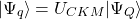efficient. The first law does not exclude the possibility of constructing a perfect engine, but the second law forbids it.

(a) A “perfect heat engine” converts all input heat into work. (b) A “perfect refrigerator” transports heat from a cold reservoir to a hot reservoir without work input. Neither of these devices is achievable in reality.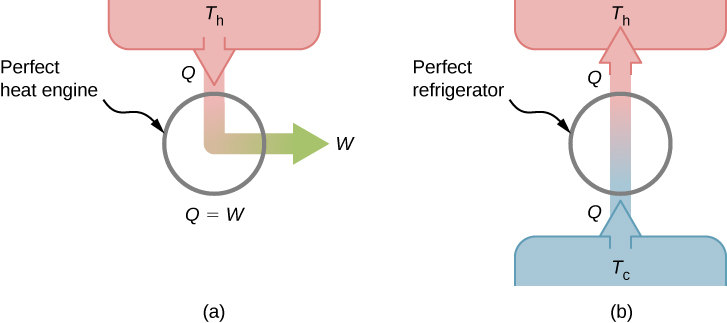We can show that the Kelvin statement is equivalent to the Clausius statement if we view the two objects in the Clausius statement as a cold reservoir and a hot reservoir. Thus, the Clausius statement becomes: It is impossible to construct a refrigerator that transfers heat from a cold reservoir to a hot reservoir without aid from an external source. The Clausius statement is related to the everyday observation that heat never flows spontaneously from a cold object to a hot object. Heat transfer in the direction of increasing temperature always requires some energy input. A “perfect refrigerator,” shown in (Figure)(b), which works without such external aid, is impossible to construct.

To prove the equivalence of the Kelvin and Clausius statements, we show that if one statement is false, it necessarily follows that the other statement is also false. Let us first assume that the Clausius statement is false, so that the perfect refrigerator of (Figure)(b) does exist. The refrigerator removes heat Q from a cold reservoir at a temperature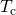and transfers all of it to a hot reservoir at a temperature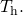Now consider a real heat engine working in the same temperature range. It extracts heat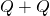from the hot reservoir, does work W, and discards heat Q to the cold reservoir. From the first law, these quantities are related by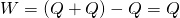.

Suppose these two devices are combined as shown in (Figure). The net heat removed from the hot reservoir is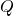, no net heat transfer occurs to or from the cold reservoir, and work W is done on some external body. Since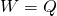, the combination of a perfect refrigerator and a real heat engine is itself a perfect heat engine, thereby contradicting the Kelvin statement. Thus, if the Clausius statement is false, the Kelvin statement must also be false.

Combining a perfect refrigerator and a real heat engine yields a perfect heat engine because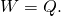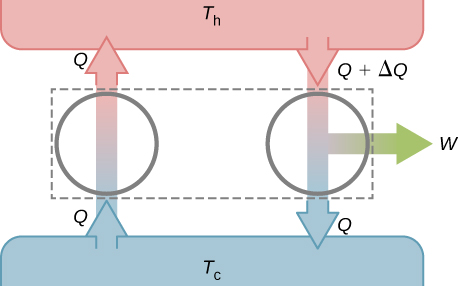Using the second law of thermodynamics, we now prove two important properties of heat engines operating between two heat reservoirs. The first property is that any reversible engine operating between two reservoirs has a greater efficiency than any irreversible engine operating between the same two reservoirs.

The second property to be demonstrated is that all reversible engines operating between the same two reservoirs have the same efficiency. To show this, we start with the two engines D and E of (Figure)(a), which are operating between two common heat reservoirs at temperatures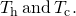First, we assume that D is a reversible engine and that E is a hypothetical irreversible engine that has a higher efficiency than D. If both engines perform the same amount of work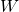per cycle, it follows from (Figure) that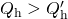. It then follows from the first law that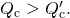(a) Two uncoupled engines D and E working between the same reservoirs. (b) The coupled engines, with D working in reverse.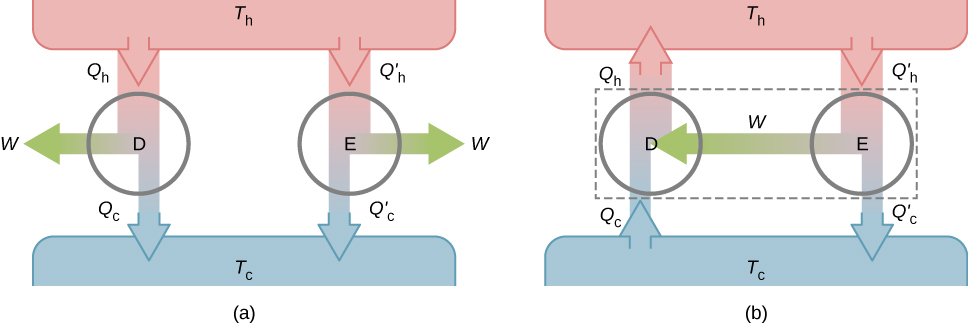Suppose the cycle of D is reversed so that it operates as a refrigerator, and the two engines are coupled such that the work output of E is used to drive D, as shown in (Figure)(b). Sinceand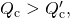the net result of each cycle is equivalent to a spontaneous transfer of heat from the cold reservoir to the hot reservoir, a process the second law does not allow. The original assumption must therefore be wrong, and it is impossible to construct an irreversible engine such that E is more efficient than the reversible engine D.

Now it is quite easy to demonstrate that the efficiencies of all reversible engines operating between the same reservoirs are equal. Suppose that D and E are both reversible engines. If they are coupled as shown in (Figure)(b), the efficiency of E cannot be greater than the efficiency of D, or the second law would be violated. If both engines are then reversed, the same reasoning implies that the efficiency of D cannot be greater than the efficiency of E. Combining these results leads to the conclusion that all reversible engines working between the same two reservoirs have the same efficiency.

Check Your Understanding What is the efficiency of a perfect heat engine? What is the coefficient of performance of a perfect refrigerator?

A perfect heat engine would have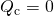, which would lead to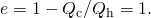A perfect refrigerator would need zero work, that is,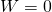, which leads to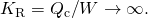Check Your Understanding Show that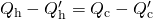for the hypothetical engine of (Figure)(b).

From the engine on the right, we have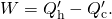From the refrigerator on the right, we have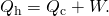Thus,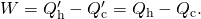### Summary

• The Kelvin statement of the second law of thermodynamics: It is impossible to convert the heat from a single source into work without any other effect.
• The Kelvin statement and Clausius statement of the second law of thermodynamics are equivalent.

### Conceptual Questions

In the text, we showed that if the Clausius statement is false, the Kelvin statement must also be false. Now show the reverse, such that if the Kelvin statement is false, it follows that the Clausius statement is false.

If we combine a perfect engine and a real refrigerator with the engine converting heat Q from the hot reservoir into work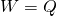to drive the refrigerator, then the heat dumped to the hot reservoir by the refrigerator will be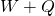, resulting in a perfect refrigerator transferring heatfrom the cold reservoir to hot reservoir without any other effect.

Why don’t we operate ocean liners by extracting heat from the ocean or operate airplanes by extracting heat from the atmosphere?

Discuss the practical advantages and disadvantages of heat pumps and electric heating.

Heat pumps can efficiently extract heat from the ground to heat on cooler days or pull heat out of the house on warmer days. The disadvantage of heat pumps are that they are more costly than alternatives, require maintenance, and will not work efficiently when temperature differences between the inside and outside are very large. Electric heating is much cheaper to purchase than a heat pump; however, it may be more costly to run depending on the electric rates and amount of usage.

The energy output of a heat pump is greater than the energy used to operate the pump. Why doesn’t this statement violate the first law of thermodynamics?

Speculate as to why nuclear power plants are less efficient than fossil-fuel plants based on temperature arguments.

A nuclear reactor needs to have a lower temperature to operate, so its efficiency will not be as great as a fossil-fuel plant. This argument does not take into consideration the amount of energy per reaction: Nuclear power has a far greater energy output than fossil fuels.

An ideal gas goes from state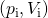to state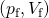when it is allowed to expand freely. Is it possible to represent the actual process on a pV diagram? Explain.

### Glossary

Kelvin statement of the second law of thermodynamics
it is impossible to convert the heat from a single source into work without any other effect
perfect engine
engine that can convert heat into work withefficiency
perfect refrigerator (heat pump)
refrigerator (heat pump) that can remove (dump) heat without any input of work